Grassmann.jl⟨Leibniz-Grassmann-Clifford-Hestenes⟩ differential geometric algebra / multivector simplicial complex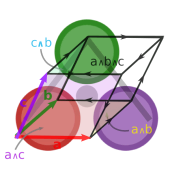The Grassmann.jl package provides tools for doing computations based on multi-linear algebra, differential geometry, and spin groups using the extended tensor algebra known as Leibniz-Grassmann-Clifford-Hestenes geometric algebra. Combinatorial products included are ∧, ∨, ⋅, *, ⋆, ', ~, d, ∂ (which are the exterior, regressive, inner, and geometric products; along with the Hodge star, adjoint, reversal, differential and boundary operators). The kernelized operations are built up from composite sparse tensor products and Hodge duality, with high dimensional support for up to 62 indices using staged caching and precompilation. Code generation enables concise yet highly extensible definitions. The DirectSum.jl multivector parametric type polymorphism is based on tangent bundle vector spaces and conformal projective geometry to make the dispatch highly extensible for many applications. Additionally, the universal interoperability between different sub-algebras is enabled by AbstractTensors.jl, on which the type system is built.

This Grassmann package for the Julia language was created by github.com/chakravala for mathematics and computer algebra research with differential geometric algebras. These projects and repositories were started entirely independently and are available as free software to help spread the ideas to a wider audience. Please consider donating to show your thanks and appreciation to this project at liberapay, GitHub Sponsors, Patreon, Tidelift, Bandcamp or contribute (documentation, tests, examples) in the repositories.

TensorAlgebra design, Manifold code generation

Mathematical foundations and definitions specific to the Grassmann.jl implementation provide an extensible platform for computing with geometric algebra at high dimensions, along with the accompanying support packages. The design is based on the TensorAlgebra abstract type interoperability from AbstractTensors.jl with a TensorBundle parameter from DirectSum.jl. Abstract tangent vector space type operations happen at compile-time, resulting in a differential conformal geometric algebra of hyper-dual multivector forms.

• DirectSum.jl: Abstract tangent bundle vector space types (unions, intersections, sums, etc.)
• AbstractTensors.jl: Tensor algebra abstract type interoperability with vector bundle parameter
• Grassmann.jl: ⟨Leibniz-Grassmann-Clifford-Hestenes⟩ differential geometric algebra of multivector forms
• Leibniz.jl: Derivation operator algebras for tensor fields
• Reduce.jl: Symbolic parser generator for Julia expressions using REDUCE algebra term rewriter

Mathematics of Grassmann can be used to study unitary groups used in quantum computing by building efficient computational representations of their algebras. Applicability of the Grassmann computational package not only maps to quantum computing, but has the potential of impacting countless other engineering and scientific computing applications. It can be used to work with automatic differentiation and differential geometry, algebraic forms and invariant theory, electric circuits and wave scattering, spacetime geometry and relativity, computer graphics and photogrammetry, and much more.

using Grassmann, Makie; @basis S"∞+++"
streamplot(vectorfield(exp((π/4)*(v12+v∞3)),V(2,3,4),V(1,2,3)),-1.5..1.5,-1.5..1.5,-1.5..1.5,gridsize=(10,10))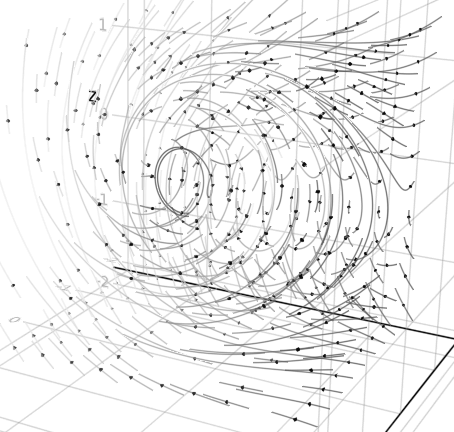Thus, computations involving fully general rotational algebras and Lie bivector groups are possible with a full trigonometric suite. Conformal geometric algebra is possible with the Minkowski plane, based on the null-basis. In general, multivalued quantum logic is enabled by the ∧,∨,⋆ Grassmann lattice. Mixed-symmetry algebra with Leibniz.jl and Grassmann.jl, having the geometric algebraic product chain rule, yields automatic differentiation and Hodge-DeRahm co/homology as unveiled by Grassmann. Most importantly, the Dirac-Clifford product yields generalized Hodge-Laplacian and the Betti numbers with Euler characteristic χ.

The Grassmann.jl package and its accompanying support packages provide an extensible platform for high performance computing with geometric algebra at high dimensions. This enables the usage of many different types of TensorAlgebra along with various Manifold parameters and interoperability for a wide range of scientific and research applications.

Requirements

Grassmann.jl is a package for the Julia language, which can be obtained from their website or the recommended method for your operating system (GNU/Linux/Mac/Windows). Go to docs.julialang.org for documentation. Availability of this package and its subpackages can be automatically handled with the Julia package manager using Pkg and Pkg.add("Grassmann") or by entering:

If you would like to keep up to date with the latest commits, instead use

which is not recommended if you want to use a stable release. When the master branch is used it is possible that some of the dependencies also require a development branch before the release. This may include (but is not limited to) the following packages:

This requires a merged version of ComputedFieldTypes at https://github.com/vtjnash/ComputedFieldTypes.jl

Interoperability of TensorAlgebra with other packages is automatically enabled by DirectSum.jl and AbstractTensors.jl.

The package is compatible via Requires.jl with Reduce.jl, SymPy.jl, SymEngine.jl, AbstractAlgebra.jl, Nemo.jl, GaloisFields.jl, LightGraphs,jl, Compose.jl, GeometryTypes,jl, Makie.jl.

Grassmann for enterprise

Sponsor this at liberapay, GitHub Sponsors, Patreon, or Bandcamp; also available as part of the Tidelift Subscription:

The maintainers of Grassmann and thousands of other packages are working with Tidelift to deliver commercial support and maintenance for the open source dependencies you use to build your applications. Save time, reduce risk, and improve code health, while paying the maintainers of the exact dependencies you use. Learn more.

DirectSum yields TensorBundle parametric type polymorphism ⨁

The DirectSum.jl package is a work in progress providing the necessary tools to work with an arbitrary Manifold specified by an encoding. Due to the parametric type system for the generating TensorBundle, the Julia compiler can fully preallocate and often cache values efficiently ahead of run-time. Although intended for use with the Grassmann.jl package, DirectSum can be used independently.

Let n be the rank of a Manifold{n}. The type TensorBundle{n,ℙ,g,ν,μ} uses byte-encoded data available at pre-compilation, where specifies the basis for up and down projection, g is a bilinear form that specifies the metric of the space, and μ is an integer specifying the order of the tangent bundle (i.e. multiplicity limit of Leibniz-Taylor monomials). Lastly, ν is the number of tangent variables.

The metric signature of the SubManifold{V,1} elements of a vector space V can be specified with the V"..." constructor by using + and - to specify whether the SubManifold{V,1} element of the corresponding index squares to +1 or -1. For example, S"+++" constructs a positive definite 3-dimensional TensorBundle.

julia> ℝ^3 == V"+++" == Manifold(3)
true

It is also possible to specify an arbitrary DiagonalForm having numerical values for the basis with degeneracy D"1,1,1,0", although the Signature format has a more compact representation. Further development will result in more metric types.

Declaring an additional plane at infinity is done by specifying it in the string constructor with at the first index (i.e. Riemann sphere S"∞+++"). The hyperbolic geometry can be declared by subsequently (i.e. Minkowski spacetime S"∅+++"). Additionally, the null-basis based on the projective split for confromal geometric algebra would be specified with ∞∅ initially (i.e. 5D CGA S"∞∅+++"). These two declared basis elements are interpreted in the type system.

The tangent map takes V to its tangent space and can be applied repeatedly for higher orders, such that tangent(V,μ,ν) can be used to specify μ and ν. The direct sum operator can be used to join spaces (alternatively +), and the dual space functor ' is an involution which toggles a dual vector space with inverted signature. The direct sum of a TensorBundle and its dual V⊕V' represents the full mother space V*. In addition to the direct-sum operation, several other operations are supported, such as ∪,∩,⊆,⊇ for set operations. Due to the design of the TensorBundle dispatch, these bit parametric operations enable code optimizations at compile-time.

Calling manifolds with sets of indices constructs the subspace representations. Given M(s::Int...) one can encode SubManifold{M,length(s),indexbits(s)} with induced orthogonal space, such that computing unions of submanifolds is done by inspecting the parameter s. Operations on Manifold types is automatically handled at compile time.

Interoperability for TensorAlgebra{V}

The AbstractTensors package is intended for universal interoperability of the abstract TensorAlgebra type system. All TensorAlgebra{V} subtypes have type parameter V, used to store a TensorBundle value obtained from DirectSum.jl. By itself, this package does not impose any specifications or structure on the TensorAlgebra{V} subtypes and elements, aside from requiring V to be a Manifold. This means that different packages can create tensor types having a common underlying TensorBundle structure.

The key to making the whole interoperability work is that each TensorAlgebra subtype shares a TensorBundle parameter (with all isbitstype parameters), which contains all the info needed at compile time to make decisions about conversions. So other packages need only use the vector space information to decide on how to convert based on the implementation of a type. If external methods are needed, they can be loaded by Requires when making a separate package with TensorAlgebra interoperability.

Since TensorBundle choices are fundamental to TensorAlgebra operations, the universal interoperability between TensorAlgebra{V} elements with different associated TensorBundle choices is naturally realized by applying the union morphism to operations. Some of the method names like +,-,⊗,×,⋅,* for TensorAlgebra elements are shared across different packages, with interoperability.

Additionally, a universal unit volume element can be specified in terms of LinearAlgebra.UniformScaling, which is independent of V and has its interpretation only instantiated by the context of the TensorAlgebra{V} element being operated on. The universal interoperability of LinearAlgebra.UniformScaling as a pseudoscalar element which takes on the TensorBundle form of any other TensorAlgebra element is handled globally. This enables the usage of I from LinearAlgebra as a universal pseudoscalar element.

Grassmann elements and geometric algebra Λ(V)

The Grassmann SubManifold elements vₖ and wᵏ are linearly independent vector and covector elements of V, while the Leibniz Operator elements ∂ₖ are partial tangent derivations and ϵᵏ are dependent functions of the tangent manifold. An element of a mixed-symmetry TensorAlgebra{V} is a multilinear mapping that is formally constructed by taking the tensor products of linear and multilinear maps. Higher grade elements correspond to SubManifold subspaces, while higher order function elements become homogenous polynomials and Taylor series.

Combining the linear basis generating elements with each other using the multilinear tensor product yields a graded (decomposable) tensor SubManifold ⟨w₁⊗⋯⊗wₖ⟩, where grade is determined by the number of anti-symmetric basis elements in its tensor product decomposition. The algebra is partitioned into both symmetric and anti-symmetric tensor equivalence classes. For the oriented sets of the Grassmann exterior algebra, the parity of (-1)^P is factored into transposition compositions when interchanging ordering of the tensor product argument permutations. The symmetrical algebra does not need to track this parity, but has higher multiplicities in its indices. Symmetric differential function algebra of Leibniz trivializes the orientation into a single class of index multi-sets, while Grassmann’s exterior algebra is partitioned into two oriented equivalence classes by anti-symmetry. Full tensor algebra can be sub-partitioned into equivalence classes in multiple ways based on the element symmetry, grade, and metric signature composite properties. Both symmetry classes can be characterized by the same geometric product.

Higher-order composite tensor elements are oriented-multi-sets. Anti-symmetric indices have two orientations and higher multiplicities of them result in zero values, so the only interesting multiplicity is 1. The Leibniz-Taylor algebra is a quotient polynomial ring so that ϵₖ^(μ+1) is zero. Grassmann’s exterior algebra doesn’t invoke the properties of multi-sets, as it is related to the algebra of oriented sets; while the Leibniz symmetric algebra is that of unoriented multi-sets. Combined, the mixed-symmetry algebra yield a multi-linear propositional lattice. The formal sum of equal grade elements is an oriented Chain and with mixed grade it is a MultiVector simplicial complex. Thus, various standard operations on the oriented multi-sets are possible including ∪,∩,⊕ and the index operation , which is symmetric difference.

By virtue of Julia’s multiple dispatch on the field type 𝕂, methods can specialize on the dimension n and grade G with a TensorBundle{N} via the TensorAlgebra{V} subtypes, such as SubManifold{V,G}, Simplex{V,G,B,𝕂}, Chain{V,G,𝕂}, SparseChain{V,G,𝕂}, MultiVector{V,𝕂}, and MultiGrade{V,G} types.

The elements of the DirectSum.Basis can be generated in many ways using the SubManifold elements created by the @basis macro,

julia> using Grassmann; @basis ℝ'⊕ℝ^3 # equivalent to basis"-+++"
(⟨-+++⟩, v, v₁, v₂, v₃, v₄, v₁₂, v₁₃, v₁₄, v₂₃, v₂₄, v₃₄, v₁₂₃, v₁₂₄, v₁₃₄, v₂₃₄, v₁₂₃₄)

As a result of this macro, all of the SubManifold{V,G} elements generated by that TensorBundle become available in the local workspace with the specified naming. The first argument provides signature specifications, the second argument is the variable name for the TensorBundle, and the third and fourth argument are the the prefixes of the SubManifold vector names (and covector basis names). By default, V is assigned the TensorBundle and v is the prefix for the SubManifold elements.

It is entirely possible to assign multiple different bases with different signatures without any problems. In the following command, the @basis macro arguments are used to assign the vector space name to S instead of V and basis elements to b instead of v, so that their local names do not interfere. Alternatively, if you do not wish to assign these variables to your local workspace, the versatile DirectSum.Basis constructors can be used to contain them, which is exported to the user as the method Λ(V).

The parametric type formalism in Grassmann is highly expressive to enable the pre-allocation of geometric algebra computations for specific sparse-subalgebras, including the representation of rotational groups, Lie bivector algebras, and affine projective geometry.

Together with LightGraphs,jl, GraphPlot.jl, Cairo.jl, Compose.jl it is possible to convert Grassmann numbers into graphs.

using Grassmann, Compose # environment: LightGraphs, GraphPlot
x = Λ(ℝ^7).v123
Grassmann.graph(x+!x)
draw(PDF("simplex.pdf",16cm,16cm),x+!x)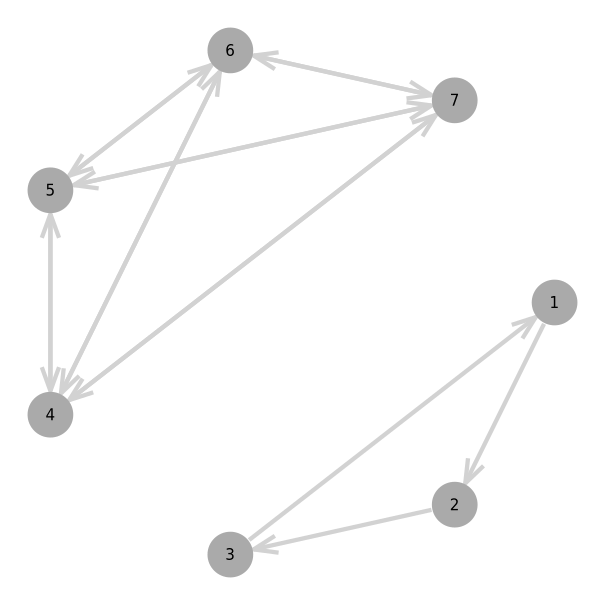Due to GeometryTypes,jl Point interoperability, plotting and visualizing with Makie.jl is easily possible. For example, the vectorfield method creates an anonymous Point function that applies a versor outermorphism:

using Grassmann, Makie
basis"2" # Euclidean
streamplot(vectorfield(exp(π*v12/2)),-1.5..1.5,-1.5..1.5)
streamplot(vectorfield(exp((π/2)*v12/2)),-1.5..1.5,-1.5..1.5)
streamplot(vectorfield(exp((π/4)*v12/2)),-1.5..1.5,-1.5..1.5)
streamplot(vectorfield(v1*exp((π/4)*v12/2)),-1.5..1.5,-1.5..1.5)
@basis S"+-" # Hyperbolic
streamplot(vectorfield(exp((π/8)*v12/2)),-1.5..1.5,-1.5..1.5)
streamplot(vectorfield(v1*exp((π/4)*v12/2)),-1.5..1.5,-1.5..1.5)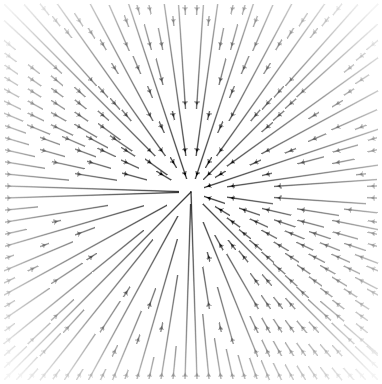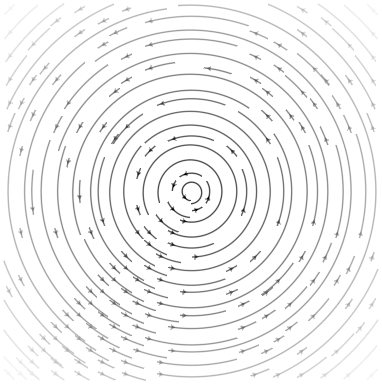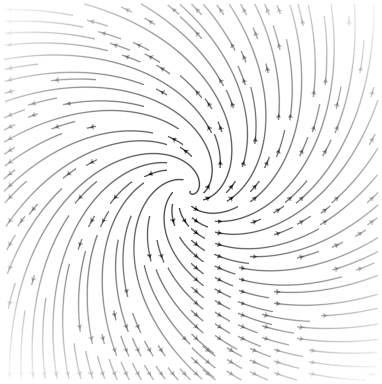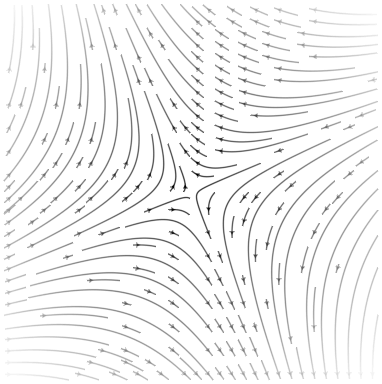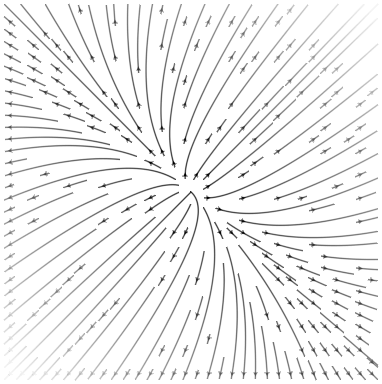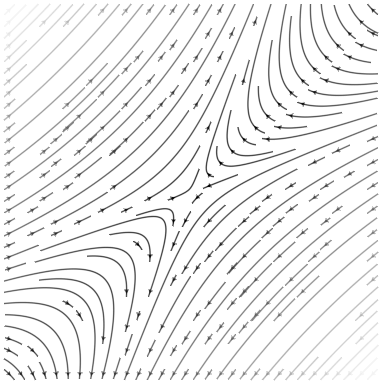using Grassmann, Makie
@basis S"∞+++"
f(t) = (↓(exp(π*t*((3/7)*v12+v∞3))>>>↑(v1+v2+v3)))
lines(V(2,3,4).(points(f)))
@basis S"∞∅+++"
f(t) = (↓(exp(π*t*((3/7)*v12+v∞3))>>>↑(v1+v2+v3)))
lines(V(3,4,5).(points(f)))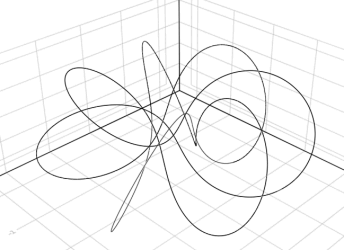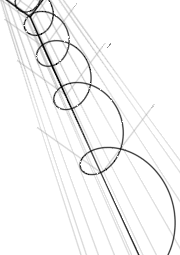using Grassmann, Makie; @basis S"∞+++"
streamplot(vectorfield(exp((π/4)*(v12+v∞3)),V(2,3,4)),-1.5..1.5,-1.5..1.5,-1.5..1.5,gridsize=(10,10))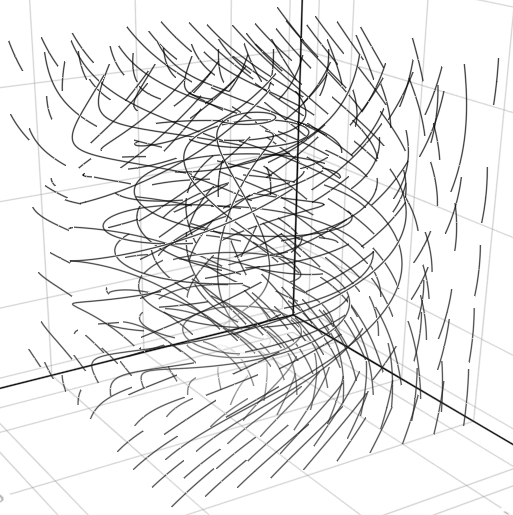using Grassmann, Makie; @basis S"∞+++"
f(t) = ↓(exp(t*v∞*(sin(3t)*3v1+cos(2t)*7v2-sin(5t)*4v3)/2)>>>↑(v1+v2-v3))
lines(V(2,3,4).(points(f)))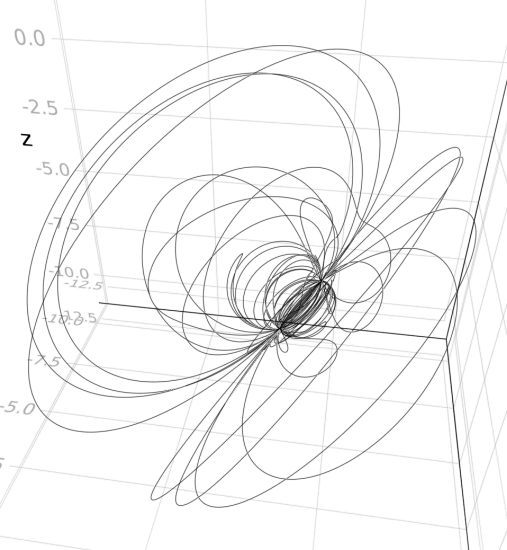using Grassmann, Makie; @basis S"∞+++"
f(t) = ↓(exp(t*(v12+0.07v∞*(sin(3t)*3v1+cos(2t)*7v2-sin(5t)*4v3)/2))>>>↑(v1+v2-v3))
lines(V(2,3,4).(points(f)))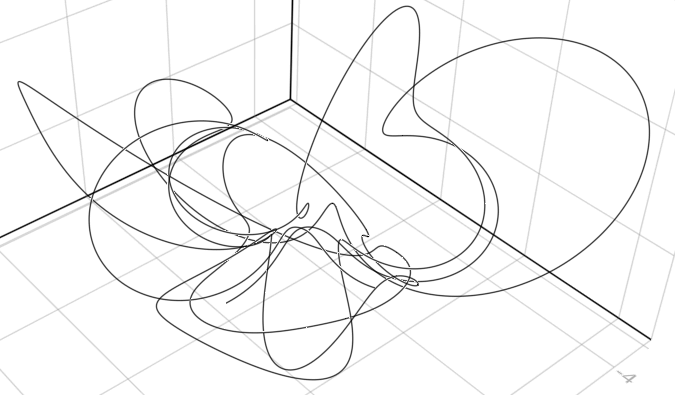Approaching ∞ dimensions with SparseBasis and ExtendedBasis

In order to work with a TensorAlgebra{V}, it is necessary for some computations to be cached. This is usually done automatically when accessed. Staging of precompilation and caching is designed so that a user can smoothly transition between very high dimensional and low dimensional algebras in a single session, with varying levels of extra caching and optimizations. The parametric type formalism in Grassmann is highly expressive and enables pre-allocation of geometric algebra computations involving specific sparse subalgebras, including the representation of rotational groups.

It is possible to reach Simplex elements with up to N=62 vertices from a TensorAlgebra having higher maximum dimensions than supported by Julia natively. The 62 indices require full alpha-numeric labeling with lower-case and capital letters. This now allows you to reach up to 4,611,686,018,427,387,904 dimensions with Julia using Grassmann. Then the volume element is

v₁₂₃₄₅₆₇₈₉₀abcdefghijklmnopqrstuvwxyzABCDEFGHIJKLMNOPQRSTUVWXYZ

Full MultiVector allocations are only possible for N≤22, but sparse operations are also available at higher dimensions. While DirectSum.Basis{V} is a container for the TensorAlgebra generators of V, the DirectSum.Basis is only cached for N≤8. For the range of dimensions 8<N≤22\$, the DirectSum.SparseBasis type is used.

julia> Λ(22)
DirectSum.SparseBasis{⟨++++++++++++++++++++++⟩,4194304}(v, ..., v₁₂₃₄₅₆₇₈₉₀abcdefghijkl)

This is the largest SparseBasis that can be generated with Julia, due to array size limitations.

To reach higher dimensions with N>22, the DirectSum.ExtendedBasis type is used. It is suficient to work with a 64-bit representation (which is the default). And it turns out that with 62 standard keyboard characters, this fits nicely. At 22 dimensions and lower there is better caching, with further extra caching for 8 dimensions or less. Thus, the largest Hilbert space that is fully reachable has 4,194,304 dimensions, but we can still reach out to 4,611,686,018,427,387,904 dimensions with the ExtendedBasis built in. Full MultiVector elements are not representable when ExtendedBasis is used, but the performance of the SubManifold and sparse elements should be just as fast as for lower dimensions for the current SubAlgebra and TensorAlgebra types. The sparse representations are a work in progress to be improved with time.